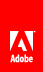# execCalculate

Executes any scripts on the calculate event of the specified object, and any child objects.

Ensure that you do not inadvertently execute this method with a larger scope than is necessary. Depending on the nature of your scripts, the calculate event could trigger multiple times in response to a single method execution, and could trigger the calculate events of other objects if the value of those objects changes because of the execution of any script.

#### Syntax

`Reference_Syntax.execCalculate()`

None

Empty

XFA 2.1

#### JavaScript

 `xfa.form.execCalculate();` Executes the scripts contained on the calculate event for all objects on the form. `Subform1.execCalculate();` Executes the scripts contained on the calculate event of the object named Subform1, and for any objects contained within the subform. `TextField1.execCalculate();` Executes the scripts contained on the calculate event of the object named TextField1.

#### FormCalc

 `xfa.form.execCalculate()` Executes the scripts contained on the calculate event for all objects on the form. `Subform1.execCalculate()` Executes the scripts contained on the calculate event of the object named Subform1, and for any objects contained within the subform. `TextField1.execCalculate()` Executes the scripts contained on the calculate event of the object named TextField1.// Ethnio survey code removed